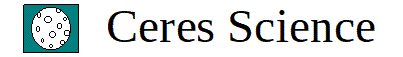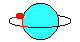For Windows - Version 2022Welcome to the High School Physics Applications. We designed several applications of great value to physics teachers and students. They include a dynamic graphical tutorial in geometrical optics, and several tools that can help physics teachers deliver motivating lessons that will keep the students engaged. The applications available are show below. Links to other pages in Ceres Science are found at the bottom of this page.

Geometrical Optics Graphical Tutorial (6)

Refraction and Reflection
Converging Lenses
Diverging Lenses
Concave Mirrors
Convex Mirrors
Geometrical Optics Graphical Tutorial

The Optics Equation

Galileo's Law of Falling Bodies (3)

Galileo I
Galileo II
Galileo III

Projectile Motion (3)

Projectile Motion I
Projectile Motion II
Projectile Motion III

Kinematics Equations

Kinematics Tutorial

Formation of Sound Beats

Relativity

the product and where to find it in our TpT store.

Geometrical Optics Graphical TutorialRefraction and Reflection Tutorial
The tutorial covers the following concepts:
Snell’s Law
Behavior of light rays when they enter a medium of greater optical density
Behavior of light rays when they enter a medium of smaller optical density
Critical angle
Behavior of light rays when they enter a converging lens
Behavior of light rays when they strike a concave mirror
Link to the Refraction and Reflection Graphical Tutorial in TpT         Cost: \$21.95

Converging Lenses
The tutorial covers the following concepts:
Case 1
Case 2
Case 3
Case 4
Case 5
Case 6
Theory of Converging Lenses
Link to Converging Lenses Graphical Tutorial in TpT         Cost: \$21.95

Diverging Lenses
The tutorial covers the following concepts:
Single Case
Theory of Diverging Lenses
Link to Diverging Lenses Graphical Tutorial in TpT         Cost: \$10.95

Concave Mirrors
The tutorial covers the following:
Case 1
Case 2
Case 3
Case 4
Case 5
Case 6
Theory of Concave Mirrors
Link to Concave Mirrors Graphical Tutorial in TpT         Cost: \$21.95

Convex Mirrors
The tutorial covers the following concepts:
Single Case
Theory of Convex Mirrors
Link to Convex Mirrors Graphical Tutorial in TpT         Cost: \$10.95

Geometrical Optics Graphical Tutorial
The tutorial covers the following topics:
Index of Refraction
Snell’s Law
Critical Angle
Refraction
Reflection
Converging Lenses (Case II)
Concave Mirrors (Case II)
Diverging Lenses
Convex Mirrors
Magnification
The Optics Equation
Real and Virtual Images
Link to the Geometrical Optics Graphical Tutorial in TpT         Cost: \$29.95

The Optics Equation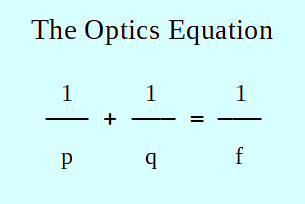This application is completely dedicated to the use of the optics equation. Any values can be entered for p, q, and f. After two values are entered, the application will indicate the properties of the image produced, what is the magnification, and the type of lens or mirror involved. It is an excellent application to practice problem solving and master geometrical optics.

Link to the Optics Equation Problem Solving Application in TpT         Cost: \$19.95

Galileo's Law of Falling Bodies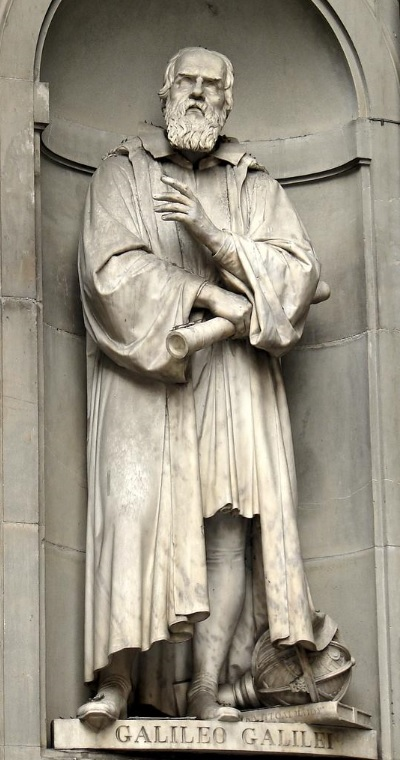There are three Galileo's Law of Falling Bodies applications. They have unique designs and are intended for physics students to learn, and for physics teachers to teach kinematics in a motivating way. Each of them presents hundreds of random numerical problems for the students to solve mentally. There is no need to use calculators. They are great activities to solve kinematics problems mentally without using equations. Instead, principles of the Law of Falling Bodies, which is a marvelous tool, need to be applied. More detailed descriptions are shown in the following TpT links.

Galileo's Law of Falling Bodies 1
There are six different types of numerical exercises. In all problems, the falling bodies start from rest.
Link to Galileo's Law of falling Bodies 1 in TpT         Cost: \$14.95

Galileo's Law of Falling Bodies 2
It incorporates four different types of exercises. In all problems, all bodies are initially thrown vertically downward.
Link to Galileo's Law of falling Bodies 2 in TpT         Cost: \$14.95

Galileo's Law of Falling Bodies 3
It incorporates four different types of exercises. In all problems, all bodies are initially thrown vertically upward.
Link to Galileo's Law of falling Bodies 3 in TpT         Cost: \$14.95

Projectile MotionThere are three Projectile Motion applications.They were designed for students to practice problem solving, and for teachers to use them as motivating teaching tools. All three application have a unique and simple design. They presents random numerical problems for the students to solve without writing anything. Some problems can be solved mentally, while others require the use of calculators. Most exercises take 30 seconds or less to solve. They include finding the range, horizontal velocity, vertical velocity, speed, height, and linear acceleration. More detailed descriptions are shown in the following TpT links.

Projectile Motion 1
In all problems, the projectiles are initially ejected horizontally.
Link to Projectile Motion 1 in TpT         Cost: \$14.95

Projectile Motion 2
In all problems, the projectiles are initially thrown above the horizontal.
Link to Projectile Motion 2 in TpT         Cost: \$14.95

Projectile Motion 3
In all problems, the projectiles are initially thrown below the horizonatl.
Link to Projectile Motion 3 in TpT         Cost: \$14.95

Kinematics Equations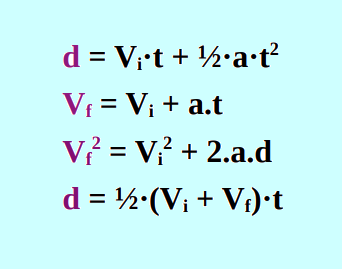The Kinematics Equations application is an excellent tool to practice kinematics problem solving. The students have the opportunity of solving 16 different types of problems. They will gain experience in using all four basic kinematics equations.

After the student indicates what type of exercise will be solved, the computer will request that information for three different variables be entered. Subsequently, the computer will estimate the correct value for the fourth variable. Only the SI System of Units is used in this application.

Link to Kinematics Equations in TpT         Cost: \$14.95

Kinematics Tutorial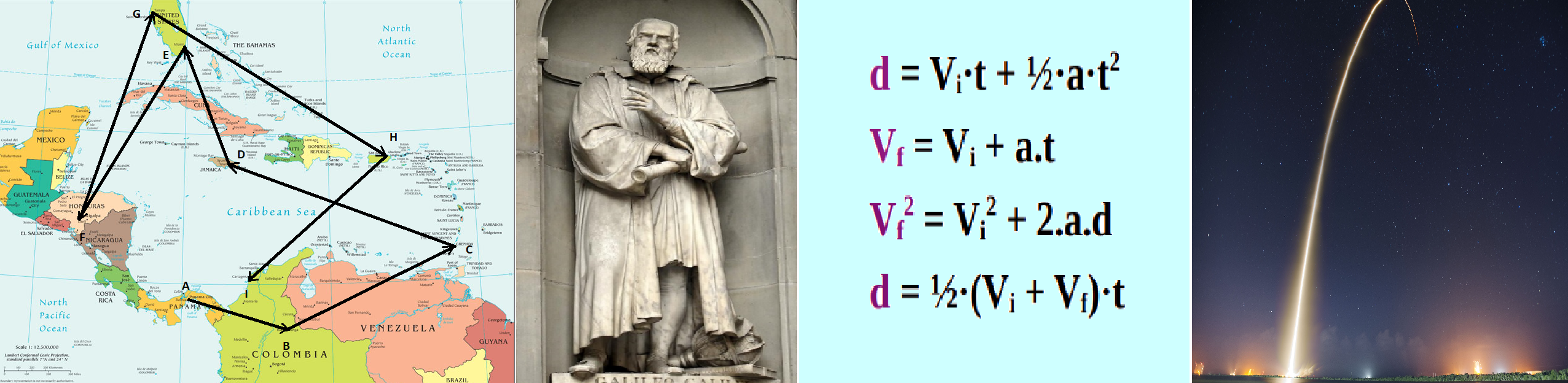This Kinematics Tutorial was designed for Honors and regular high school physics students. The tutorial covers the following topics:

Vectors
Uniform Motion in a Straight Line
Uniformly Accelerated Motion
Galileo’s Law of Falling Bodies
Galileo’s Law of Odd Numbers
Kinematic s Equations
Projectile Motion

Every concept from vectors to projectile motion is explained using simple language and several examples. After the students go over the 48 screens the tutorial incorporates, they will have solid knowledge of kinematics and will be ready for problem solving practice in most of the chapter.

Link to the Kinematics Tutorial Application in TpT         Cost: \$19.95

Formation of Sound Beats - Addition of Waves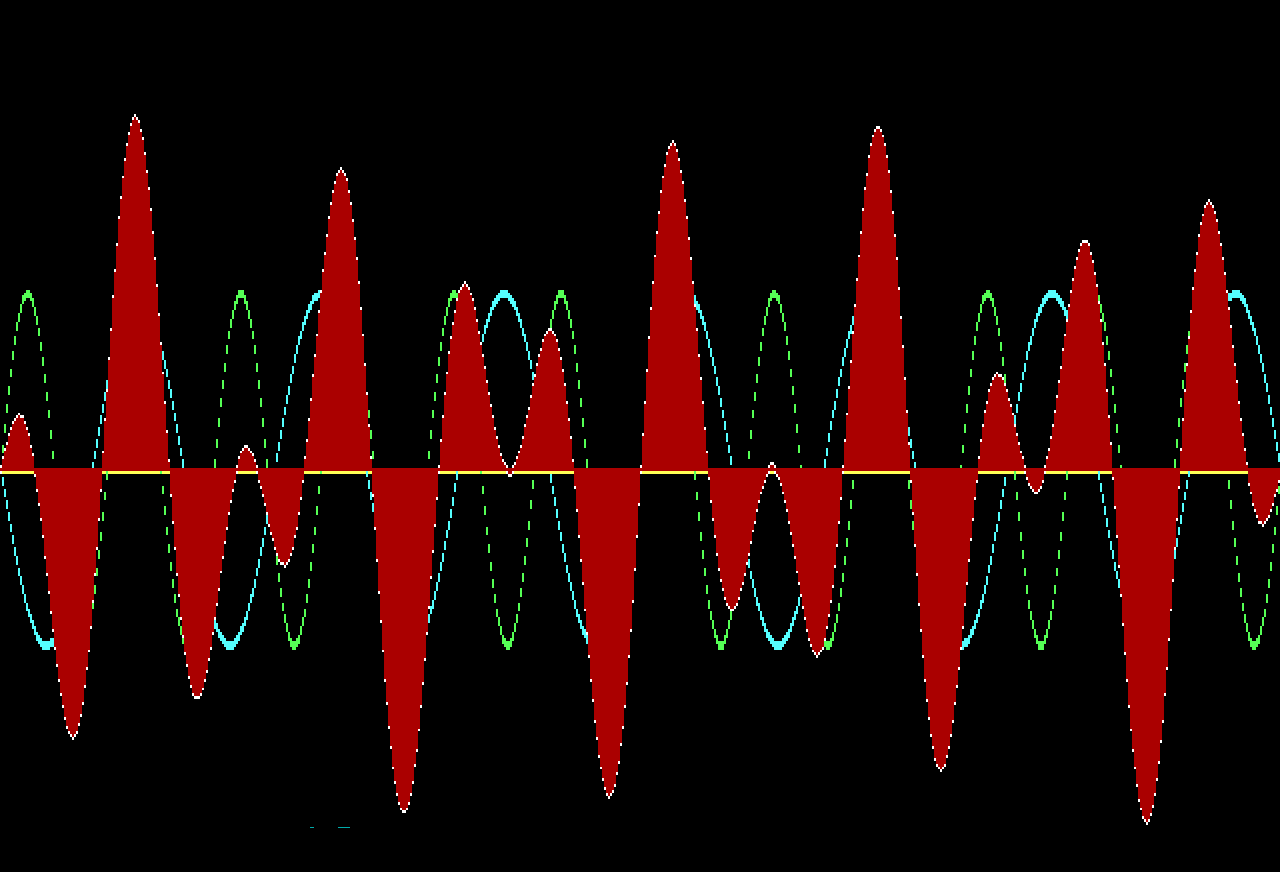This application shows the formation of beats when sound waves interfere and combine. In other words, when waves are added. It can be used by physics teachers during the study of waves. The students will enjoy the graphics and the great variety of beats formed. A good thing is that many different frequencies and amplitudes can be entered, forming thousands of different beats patterns.

Link to the Formation of Beats - Addition of Waves Application in TpT         Cost: \$14.95

Relativity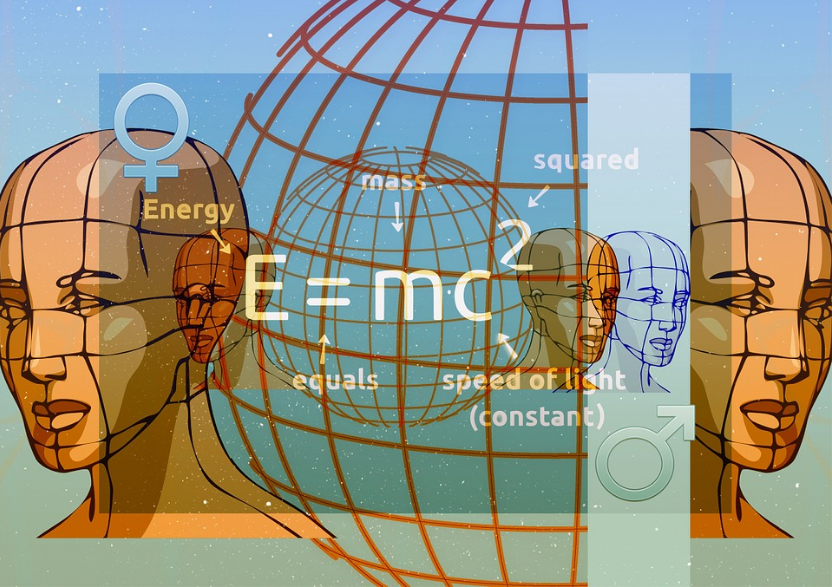Relativity 1
This physics application shows the time dilation, the mass expansion, and the length contraction when one student in a hypothetical journey travels in space at speeds near the speed of light. The application will assume the following information for one student:

Mass of the student = 60 kg
Age of the student = 18 years
Length of the spacecraft = 150 m
Year the student left planet Earth = 2,022

The student will return to Earth after 20 years, as indicated by a clock in the spacecraft.

By just pressing each time the space bar, starting at 0.1c and increasing gradually all the way to 0.999999998c, the computer will show:

Mass of the student while traveling in spacecraft
Length of the spacecraft while moving in space
Age of the student when returning to Earth
Years passed on planet Earth since the student left
Current year in Planet Earth when the student returned
Link to Relativity 1 in TpT         Cost: \$14.95

Relativity 2
The application Relativity 2 shows the time dilation, the mass expansion, and the length contraction when one student in a hypothetical journey travels in space at speeds near the speed of light. The application will request the following information to be entered:

Mass of the student
Age of the student
Speed of the spacecraft (from 0.1c to 0.999999998c)
Year the student left planet Earth

The student will return after 1,000 years have passed on planet Earth. The computer will find the following:

Mass of the student while traveling in spacecraft
Length of the spacecraft while moving in space
Age of the student after 1,000 years have passed on planet Earth
Year in Planet Earth when the student returned
Link to Relativity 2 in TpT         Cost: \$14.95

Home Page      Physics Worksheets      Chemistry      Biology      Physical Science      Links to Ceres Science in TpT      AP Physics 1 Projects
Earth & Space Science      Physics Test Generators       Physics Video Exams      Anatomy         Balsa Wood Bridges      Electric Motors
Computer Science Worksheets       Health Science        Zoology        View & Play Activities       Free Materials       Boards in Pinterest
BASIC Language       Physics Applications        Chemistry Applications        Physics Lessons       All Science Products       To Facebook